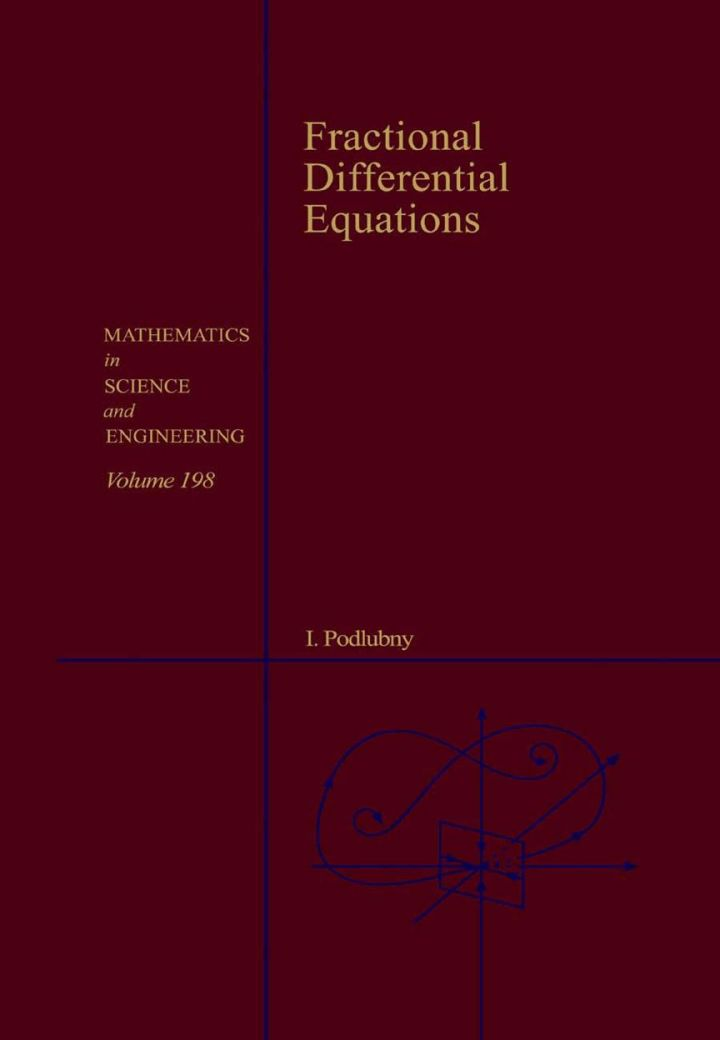# Fractional Differential Equations: An Introduction to Fractional Derivatives, Fractional Differential Equations, to Methods of Their Solution and Some of Their Applications

## Fractional Differential Equations: An Introduction to Fractional Derivatives, Fractional Differential Equations, to Methods of Their Solution and Some of Their Applications

By Podlubny, Igor

### Rent or Buy eTextbook

Expires on Apr 16th, 2022
\$126

Publisher List Price: \$129.99
Savings: \$3.99

This book is a landmark title in the continuous move from integer to non-integer in mathematics: from integer numbers to real numbers, from factorials to the gamma function, from integer-order models to models of an arbitrary order. For historical reasons, the word 'fractional' is used instead of the word 'arbitrary'.
This book is written for readers who are new to the fields of fractional derivatives and fractional-order mathematical models, and feel that they need them for developing more adequate mathematical models.
In this book, not only applied scientists, but also pure mathematicians will find fresh motivation for developing new methods and approaches in their fields of research.
A reader will find in this book everything necessary for the initial study and immediate application of fractional derivatives fractional differential equations, including several necessary special functions, basic theory of fractional differentiation, uniqueness and existence theorems, analytical numerical methods of solution of fractional differential equations, and many inspiring examples of applications.

Key Features
* A unique survey of many applications of fractional calculus
* Presents basic theory
* Includes a unified presentation of selected classical results, which are important for applications
* Provides many examples
* Contains a separate chapter of fractional order control systems, which opens new perspectives in control theory
* The first systematic consideration of Caputo's fractional derivative in comparison with other selected approaches
* Includes tables of fractional derivatives, which can be used for evaluation of all considered types of fractional derivatives

Subject: Mathematics & Statistics -> Mathematics -> Mathematics GeneralPublisher: Elsevier S & T 10/1998
Imprint: Academic Press
Language: English
Length: 340 pages

ISBN 10: 0125588402
ISBN 13: 9780125588409
Print ISBN: 9780125588409

Live Chats